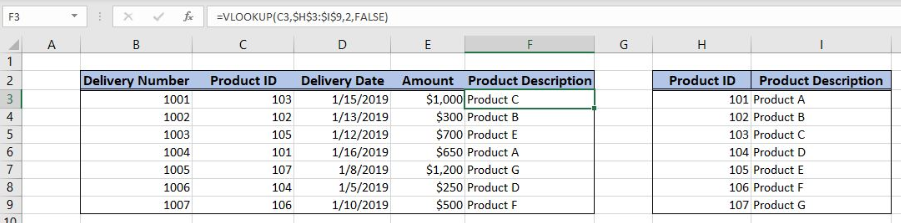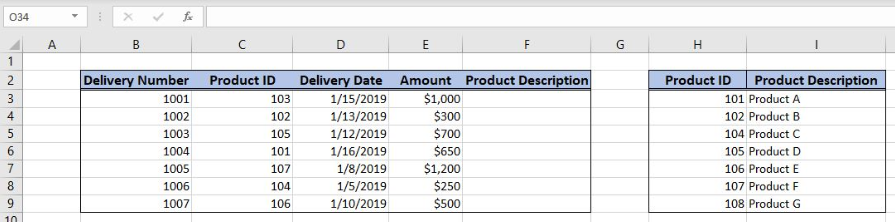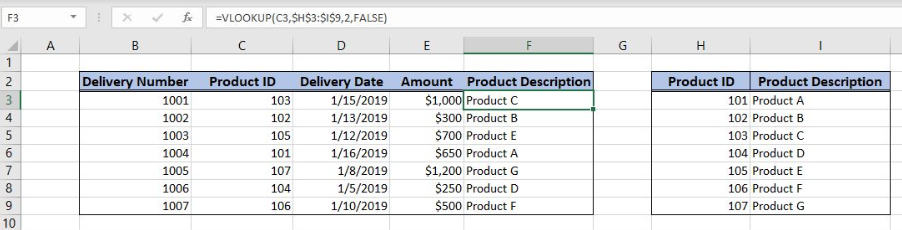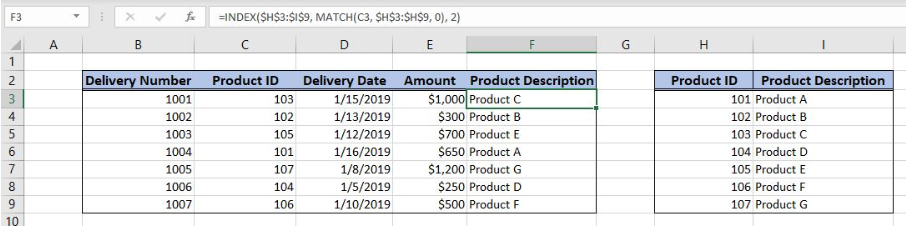Get instant live expert help with Excel or Google Sheets“My Excelchat expert helped me in less than 20 minutes, saving me what would have been 5 hours of work!”

#### Post your problem and you’ll get expert help in seconds.

Your message must be at least 40 characters
Our professional experts are available now. Your privacy is guaranteed.

# What Are the Different Ways to Do Lookups in Excel?

Excel allows us to lookup values in two ways. Using the VLOOKUP function or with INDEX and MATCH functions. The MATCH function returns a row for a value in a table, while the INDEX returns a value for that row. This step by step tutorial will assist all levels of Excel users in learning the different ways of lookups.Figure 1. The final result of the formula

## Syntax of the VLOOKUP formula

The generic formula for the VLOOKUP function is:

`=VLOOKUP(lookup_value, table_array, col_index_num, range_lookup)`

The parameters of the VLOOKUP function are:

• lookup_value – a value that we want to find in a table_array
• table_array – a range in which we want to lookup
• col_index_num – a column number in table_array from which we would like to get a value
• range_lookup – default value is FALSE. This means that we want to find an exact match for a lookup value.

## Syntax of the INDEX formula

The generic formula for the INDEX function is:

`=INDEX(array, row_num, column_num)`

The parameters of the INDEX function are:

• array – a range of cells where we want to get a data
• row_num – a number of a row in the array for which we want to get a value
• column_num – a column in the array which returns a value.

## Syntax of the MATCH formula

The generic formula for the MATCH function is:

`=MATCH(lookup_value, lookup_array, [match_type])`

The parameters of the MATCH function are:

• lookup_value – a value which we want to find in the lookup_array
• lookup_array – the array where we want to find a value
• [match_type] – a type of match. We put 0 which is an exact match.

## Setting up Our Data for the Examples

Our table consists of 5 columns: “Delivery Number” (column B), “Product ID” (column C), “Delivery Date” (column D), “Amount” (column E) and “Product Description” (column F).

In column F, we want to populate a Product Description from the Lookup table in the range H3:I9. This table consists of 2 columns: “Product ID” (column H) and “Product Description” (column I). Two tables are joined by the columns “Product ID”.Figure 2. Data that we will use in the example

## Using the VLOOKUP Formula

In the first example, we will use the VLOOKUP function. We want to get a product description in the cell F3, from the lookup table H3:I9, based on the Product ID 103 in the cell C3.

The formula looks like:

`=VLOOKUP(C3, \$H\$3:\$I\$9, 2, FALSE)`

The lookup_value parameter is C3. The table_array is \$H\$3:\$I\$9. The range must be fixed, as it’s not changing when the formula is copied to the other cells. The col_index_num is 2 because we want to get a value from the second column. The range_lookup is FALSE.

To apply the VLOOKUP formula, we need to follow these steps:

• Select cell F3 and click on it
• Insert the formula: `=VLOOKUP(C3, \$H\$3:\$I\$9, 2, FALSE)`
• Press enter
• Drag the formula down to the other cells in the column by clicking and dragging the little “+” icon at the bottom-right of the cell.Figure 3. Using the VLOOKUP formula

The function returns “Product C” in F3 as this is the description for the Product ID 103 from C3.

## Using the INDEX and MATCH Formula

Now, we will do the same example using the INDEX and MATCH functions.

The formula looks like:

`=INDEX(\$H\$3:\$I\$9, MATCH(C3, \$H\$3:\$H\$9, 0), 2)`

The lookup_value parameter of the MATCH function is C3. The lookup_array is \$H\$3:\$H\$9, while the match_type is 0, as we want the exact match. The result of the MATCH function is the row_num parameter of the INDEX function. The array the range \$H\$3:\$I\$9.

To apply the formula, we need to follow these steps:

• Select cell F3 and click on it
• Insert the formula: `=INDEX(\$H\$3:\$I\$9, MATCH(C3, \$H\$3:\$H\$9, 0), 2)`
• Press enter
• Drag the formula down to the other cells in the column by clicking and dragging the little “+” icon at the bottom-right of the cell.Figure 4. Using the INDEX and MATCH Formula

Again, the result in the cell F3 is the same as in the previous example with VLOOKUP – “Product C”.

Most of the time, the problem you will need to solve will be more complex than a simple application of a formula or function. If you want to save hours of research and frustration, try our live Excelchat service! Our Excel Experts are available 24/7 to answer any Excel question you may have. We guarantee a connection within 30 seconds and a customized solution within 20 minutes.

Solution examplesindex and match with duplicates. I need to use another column as a reference, so my return value has two match the value of two things for it to return
Solved by E. H. in 60 minsI have a file with two sheets. On the 1st, I have dollars in O13and in O16 a number signifying 1-6 people. On the second workbook is a matrix that corresponds to the dollar amount AND the number of people. For example, sheet 1 O13 is \$6,000. O16 is 3. I want a formula to find \$6,000 (not higher) on sheet 2 in column A. Then I want it to compare sheet 1 O16 to the correct column (B-G representing 1-6) on sheet 2 for an exact match to place in sheet1 O17. The range of sheet1 O13 is referenced on sheet2 A5-A383, and sheet 1 O16 is referenced on sheet2 B5:G383. I have an INDEX Match but it isn't quite working, yet.
Solved by B. B. in 60 minsI need a formula to compare the data in two columns and then export the mismatched data in the 3rd column
Solved by S. Q. in 20 minsI have two lists. One is a list of part numbers. The other is a list of long text descriptions. I can use VLOOKUP with "*"&cell address"*" to find the first match. But how can I find the second and third matches? I have tried using INDEX and SHORT but the wild cards no longer find the match like they do in VLOOKUP. Can you help?
Solved by S. J. in 36 minsI filtered data in column A (it is labeled ID #) of a data set. On my next worksheet, column A is also the ID #, but it is a different data set. I want to filter the ID #'s the same for the two sheets, but how do I do that being that I am working with two different sets of data?
Solved by V. H. in 22 mins